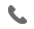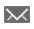•+44 7459 302492info@uplatz.com
• Register
Job Meter = High

30 Hours
Online Instructor-led Training
USD 1399 (USD 2800)
Save 50% Offer ends on 31-Dec-2023318 Learners

# Statistics 1: Introduction to ANOVA, Regression, and Logistic Regression

This introductory course is for SAS software users who perform statistical analyses using SAS/STAT software. The focus is on t tests, ANOVA, and linear regression, and includes a brief introduction to logistic regression. This course (or equivalent knowledge) is a prerequisite to many of the courses in the statistical analysis curriculum.

Learn how to
• Generate descriptive statistics and explore data with graphs.
• Perform analysis of variance and apply multiple comparison techniques.
• Perform linear regression and assess the assumptions.
• Use regression model selection techniques to aid in the choice of predictor variables in multiple regression.
• Use diagnostic statistics to assess statistical assumptions and identify potential outliers in multiple regression.
• Use chi-square statistics to detect associations among categorical variables.
• Fit a multiple logistic regression model.
• Score new data using developed models.

# Predictive Modeling Using Logistic Regression

This course covers predictive modeling using SAS/STAT software with emphasis on the LOGISTIC procedure. This course also discusses selecting variables and interactions, recoding categorical variables based on the smooth weight of evidence, assessing models, treating missing values and using efficiency techniques for massive data sets.

Learn how to
• use logistic regression to model an individual's behavior as a function of known inputs
• create effect plots and odds ratio plots using ODS Statistical Graphics
• handle missing data values
• tackle multicollinearity in your predictors
• assess model performance and compare models.
------------------------------------------------------------------------------
Target Audience

Statisticians, researchers, and business analysts who use SAS programming to generate analyses using either continuous or categorical response (dependent) variables

Modelers, analysts and statisticians who need to build predictive models, particularly models from the banking, financial services, direct marketing, insurance and telecommunications industries
------------------------------------------------------------------------------

Course Details & Curriculum

# Statistics 1: Introduction to ANOVA, Regression, and Logistic Regression

Course Overview and Review of Concepts
• Descriptive statistics.
• Inferential statistics.
• Examining data distributions.
• Obtaining and interpreting sample statistics using the UNIVARIATE procedure.
• Examining data distributions graphically in the UNIVARIATE and FREQ procedures.
• Constructing confidence intervals.
• Performing simple tests of hypothesis.
• Performing tests of differences between two group means using PROC TTEST.
ANOVA and Regression
• Performing one-way ANOVA with the GLM procedure.
• Performing post-hoc multiple comparisons tests in PROC GLM.
• Producing correlations with the CORR procedure.
• Fitting a simple linear regression model with the REG procedure.
More Complex Linear Models
• Performing two-way ANOVA with and without interactions.
• Understanding the concepts of multiple regression.
Model Building and Effect Selection
• Automated model selection techniques in PROC GLMSELECT to choose from among several candidate models.
• Interpreting and comparison of selected models.
Model Post-Fitting for Inference
• Examining residuals.
• Investigating influential observations.
• Assessing collinearit.
Model Building and Scoring for Prediction
• Understanding the concepts of predictive modeling.
• Understanding the importance of data partitioning.
• Understanding the concepts of scoring.
• Obtaining predictions (scoring) for new data using PROC GLMSELECT and PROC PLM.
Categorical Data Analysis
• Producing frequency tables with the FREQ procedure.
• Examining tests for general and linear association using the FREQ procedure.
• Understanding exact tests.
• Understanding the concepts of logistic regression.
• Fitting univariate and multivariate logistic regression models using the LOGISTIC procedure.
• Using automated model selection techniques in PROC LOGISTIC including interaction terms.
• Obtaining predictions (scoring) for new data using PROC PLM.

# Predictive Modeling Using Logistic Regression

Predictive Modeling
• analytical challenges
Fitting the Model
• parameter estimation
Preparing the Input Variables
• missing values
• categorical inputs
• variable clustering
• variable screening
• subset selection
Classifier Performance
• ROC curves and Lift charts
• optimal cutoffs
• K-S statistic
• c statistic
• profit
• evaluating a series of models
------------------------------------------------------------------------------